# Informática

Introduction

The finite element method is the most popular means of simulating an engineering system for a variety of technical disciplines.

It represents the main technique for performing stress analysis used daily by modern industry.

Its success is due to the fact that it allows to solve analysis of complex geometries once the geometry has been described and the conditions of work have been established; without this method these problems would have just an approximate solution.

This report concerns my first approach to the application of the finite element method of a stress analysis of a ball valve body.

The aim of this report is to describe the main changes to the provided VALVE_C input file and explain why they have been done to show that we have achieved a satisfactory result; more in detail, I have created different models by changing the discretization and the mesh of the model.

Thereafter a compromise must be found between having very accurate results and performing a rapid analysis.

Definition of the model

• Objectives

We aim to carry out the stress analysis of a ball valve body by using the finite element method.

The analysis is focussed in the intersection of the spherical cylindrical parts where the maximum stress concentration in expected.

The finite element method is applied in order to solve a complex geometries analysis; it is worth recalling that in stress analysis the basic equations for displacement, stress and strain are known but cannot be solved for complex geometries.

The basic concept in the finite element method is that a finite element model made of elements of simpler shapes for which the basic equations can be solved approximates the real component.

• Geometry

The valve body can be considered as a sphere with two intersecting cylinders at the ends of which two flange rings are located.

If the valve spindle is neglected and it is assumed that the opening will be somehow reinforced, then the geometry has three possible planes of symmetry.

We have done an important simplification and the model is reduced to a eighth of the original.

The following dimensions are given:

• Sphere inside radius: 95 mm

• Sphere outside radius: 105 mm

• Cylinder inside radius: 32.5 mm

• Cylinder outside radius: 47.5 mm

• Flange thickness : 20 mm

• Overal length: 200 mm

The model is created by drawing two arcs for the sphere and intersecting them with the lines that define the cylinder.

We use the LCSL command to choose the lines, break them at intersection and the LDEL command to delete the unwanted lines.

The same commands are used for creating the flange.

Then the areas are highlighted and at the end the volume is created sweeping the areas around 90° using the VDRAG command.

The valve is subjected to a static pressure of 20 bar, which is given to be constant and uniform in all internal surface of the valve; the load has the same symmetry of the valve.

Pressure is applied using the SFA command.

The weight of the valve has not been considered.

• Constraints

Rigid supports are considered in all the points located in the planes x = 0 , y = 0 ,

z = 0 ; this is done in order to keep the model firm in the places where it has been cut by symmetry planes.

• Material

Its Young's module and Poisson's coefficient define the material.

They are given as follows:

• Ex = 207000 N/m2

•  = 0.3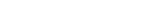Changes in the model

As mentioned before the ball valve is subjected to an internal pressure and the maximum stress is located on the inside surface around the corner.

In order to minimise the stress concentration effects we have introduced fillets in the intersection between the cylinder and the sphere, on the inside and outside surface of the valve.

Two fillets of 5 mm were created by using the LFILLT command.

The syntax is shown:

LFILLT , NL1 , NL2 , R

Where NL1 and NL2 are the lines to be filleted and R is the radius.

The inclusion of the fillet reduces the value of the stress.

Comparing the models with different fillet radii we can notice that an increase of the fillet radius reduces the stress gradient and the maximum stress but the change in radius leads to a decrease of only 1 % in stress and therefore the variation is not significant; for this reason I give account of the results only with a given radius of 5 mm.

Discretization and mesh

The process of meshing is the most complicated.

It consists of dividing the volume into brick elements of the most appropriate dimension.

There are a lot of possibilities and often there is no right or wrong choice for the correct mesh size but you have to remember that a more detailed mesh is also more expensive in terms of analysis time and computer resources and does not guarantee results of any significantly greater accuracy than a larger mesh size.

For these reasons the process requires a careful analysis in that a balance has to be found between the accuracy of the model and the effectiveness of its application.

We were required to choose a suitable mesh size from which we could carry out the analysis.

We tried two different ways to mesh the volume in order to find the best.

At the beginning the model was tested with an automatic mesh.

The syntax is shown:

ET , 1 , 45

ESIZE , 10 ( or ESIZE , 5 )

VMESH , ALL

Two meshes have been done: the first mesh has an element size of 10 mm the second mesh one of 5 mm.

Obviously the last one is more accurate than the first in particular where the stress gradient varies quite markedly (region of fillet) but it has an excess of elements in location away from the discontinuities

In order to combine the positive features of both meshes and save computer processing time since less accuracy was required in the other parts of the valve we have tested the model with a manual mesh.

This consists of using the LESIZE command that defines a variable spacing mesh over the model.

So we have divided the lines in a certain number of divisions with a spacing ratio, this allows to have a more accurate mesh around the fillet (smaller elements) and not to have too many elements through the thickness, especially because the wall thickness is thin compared with the mean radius.

This command allows you to uniformly vary the mesh size over the model and to get it more accurate in the region of fillet.

We have meshed twice: once with a brick depth of 10 mm and the second time of 5 mm.

The second one is more accurate but the process is really slow because the number of the elements and perhaps it has too many elements defined in the circunferential direction.

FIG. 1 mesh using LESIZE command FIG. 2 mesh using LESIZE command

Results

It is easy to understand that several variation of element size and mesh types were tried in order to get an evaluation of the results changes between the solutions.

We have to remember that time and money are really important in industry and it is really important to choose a solution that represents a good compromise between the two of them.

The results obtained from using size 5 are more accurate but they are not considered the best method of analysis because the computing time required to obtain the solution is considerable.

A graphical display around the area of the crotch corner showing the stress intensity gradient and mesh can be seen below.

FIG. 3 Showing the charge in stress concentration and the variable mesh size

FIG. 4 Zoom on the fillet region showing the stress intensity gradient

Hand calculation

To check the validity of the results obtained from ANSYS we should do some hand calculations.

Generally the results obtained from the verification are never the same as the Ansys solution because they are based on simply geometry while Ansys solution is based on more complex geometry but anyway you can confirm that the results are somewhere in the correct field of play.

Principal stress equations for the sphere (expressed in spherical co-ordinates)

Equation 1

Equation 2

Principal stress equations for a cylinder (expressed in cylindrical co-ordinates)

Equation 3

Equation 4

Equation 5

Where : Ri : sphere/cylinder inner radius

You can find the values of the parameters in the paragraph `geometry'.

P is the fluid pressure inside the valve and has a value of 20 bar.

The equivalent Von Mises' stress is :

Equation 6

Using equations 1 and 2 for the sphere we get :

1 = 2 = 9.02 N/mm2 and 3 = - 0.9 N/mm2

e = 9.92 N/mm2

Using the equation 3 and 4 for the cylinder we get :

1 = 0 N/mm2 2 = 4.24 N/mm2 3 = - 0.72 N/mm2

e = 4.86 N/mm2

Therefore, the equivalent Von Mises' stress for the cylinder model is around 5 N/mm2 and around 10 N/mm2 for the sphere model.

Discussion and conclusion

From all the studies we have done we are able to draw the following conclusions:

Using the smallest possible mesh size doesn't always guarantee the best results.

SMALLER IS NOT ALWAYS BETTER

As you can notice by the results from the analysis carried out with the mesh size of 5 mm we get more accurate results but the difference is irrelevant e does not justify the long computing time and the use of computer resources.

We can say that the results obtained using LESIZE command give the best balance between accuracy and time because we are able to mesh the area around the crotch corner more finely without wasting time in further away surfaces.

We can also say that the inclusion of the fillet reduces drastically the value of the stress concentration factor but an increase in the fillet radius makes no significant difference in the value of the maximum stress in the fillet area (only a bit more than 1 N/mm2).

So I can conclude that the original fillet of 5 mm is an acceptable radius since any increase doesn't significantly effect the results.

The maximum stress intensity value found has a value approximately------N/mm2 and this is well within the yield point for steel of about 250 N/mm2.

This indicates that a material saving could be made by reducing the body thickness of the valve.

This suggestion is acceptable only if the internal pressure of the valve is not increased beyond 2 N/mm2, if this happens an other study has to be carried out.

9

Coursework 1

16429-Computer Aided Engineering Design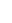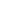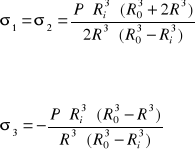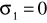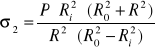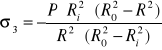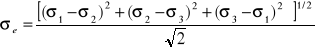Enviado por: Sergio Núnez Idioma: inglés País: España

Palabras clave:
Te va a interesar